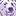# New to Qlik Sense

If you’re new to Qlik Sense, start with this Discussion Board and get up-to-speed quickly.

Announcements
On-Demand Webinar: Learn proven best practices for a smooth transition to Qlik Sense® SaaS. REGISTER
cancel
Showing results for
Did you mean:Creator

## Count of items lying in each quadrant in a scatter plot

Hi Guys,

I have created a scatter plot based on Stores and the sales by the each store. I would like to know the count of stores that lie in each quadrant. Is this possible? Please let me know.

Best Regards,

Rony

Labels (4)

• ### SaaS

17 RepliesCreator
Author

Nope. Error in set modifier expressionCreator

What is your dimension name? Store? Stores?Creator
Author

Dimension name is Location.Creator

My bad, it was missing couple parentheses

``````Count({<Location=
{"=(((avg({\$<Year={'2019'}>}ASP))/(avg({\$<Year={'2018'}>}ASP)))-1)<=0"}
>*<Location=
{"=(((avg({\$<Year={'2019'}>}Volume))/(avg({\$<Year={'2018'}>}Volume)))-1)<=0"}>}
distinct Location)``````Creator
Author

Hi Anseg,

The expression is working fine. However, the count is coming as 0. Not sure why.

RonyCreator
Author

Hi Anseg,

Below is the expression I am using right now. I have expanded the ASP formula as the one I was using earlier was wrong.The expression is correct. However, the count is still not showing. Where am I going wrong? Please advise

Also attached is a screenshot of the expression page

Count({<Location= {"=\$(=((Sum({\$<Year={"2019"}>}Value)/Sum({\$<Year={"2019"}>}Volume))/
(Sum({\$<Year={"2018"}>}Value)/Sum({\$<Year={"2018"}>}Volume))-1))
<=0"}>*<Location= {"=\$(=(Sum({\$<Year={"2019"}>}Volume)/Sum({\$<Year={"2018"}>}Volume)-1))<=0"}>} distinct Location)

RonyCreator

Okay my bad again, in my original reply I had the \$(= but they shouldn't be there so you can remove them

``````Count({<Location=
{"=((Sum({\$<Year={'2019'}>}Value)/Sum({\$<Year={'2019'}>}Volume))/(Sum({\$<Year={'2018'}>}Value)/Sum({\$<Year={'2018'}>}Volume))-1)<=0"}
>*<Location=
{"=(Sum({\$<Year={'2019'}>}Volume)/Sum({\$<Year={'2018'}>}Volume)-1)<=0"}>}
distinct Location)``````

How is this?Creator
Author

This is perfect. Thanks a lot Anseg!!😊

Best Regards,

RonyTags
Community Browser